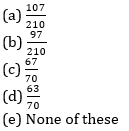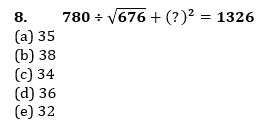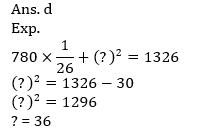# IBPS PO Quantitative Aptitude Quiz- 4

## IBPS PO Quantitative Aptitude Quiz

Quantitative aptitude measures a candidate’s numerical proficiency and problem-solving abilities. It is the most important section of almost all competitive exams. Candidates are often stymied by the complexity of Quantitative Aptitude Questions but if they practice more and more questions it will become quite easy. So, here we are providing you with the IBPS PO Quantitative Aptitude Quiz to enhance your preparation for your upcoming examination. Questions given in this IBPS PO Quantitative Aptitude Quiz are based on the most recent and the latest exam pattern. A detailed explanation for each question will be given in this IBPS PO Quantitative Aptitude Quiz. This IBPS PO Quantitative Aptitude Quiz is entirely free of charge. This IBPS PO Quantitative Aptitude Quiz will assist aspirants in achieving a good score in their upcoming examinations.

1. The probability of selection of three candidates A, B and C in an organization is 2/5, 5/6 and 4/7 respectively. Find the probability that at least one of them get selected.2. If the ratio of compound interest and principle for two years is 7 : 9 and the difference between CI and SI for same period is Rs 180. Then find the simple interest on same principle for three years at same rate ?
(a) Rs. 1440
(b) Rs. 1620
(c) Rs. 1820
(d) Rs. 1280
(e) Rs. 16403. The ratio of height and radius of a cylinder is 2 : 5 and diameter of cylinder is equal to the diagonal of square whose area is 400 m². Then find the volume of cylinder (in m³)?4. An article is sold at 40% discount on M.P. and ratio of C.P. to S.P. is 5 : 8 then what is the M.P. of article if the profit obtained on same article is Rs. 450.
(a) Rs. 1400
(b) Rs. 2400
(c) Rs. 1600
(d) Rs. 2200
(e) Rs. 20005. In how many ways a committee of six persons can be made out of five male and four females such that there is at most four males in the committee?
(a) 84
(b) 60
(c) 120
(d) 80
(e) 100Directions (6-10): What should come in place of question mark (?) in the following questions?
6. 4368 + 2158 – 596 – ? = 3421 + 1262 + 5² × 48
(a) 35
(b) 47
(c) 51
(d) 56
(e) 45

Ans. b
Exp.
? = 6526 – 596 – 4683 – 1200
? = 479. 3695.12 + 4458.02 – ? = 7592.14
(a) 562.14
(b) 661.14
(c) 561
(d) 561.14
(e) 661

Ans. c
Exp.
8153.14 – ? = 7592.14
? = 561###Click to Buy Bank MahaCombo PackageRecommended PDF’s for:

#### Most important PDF’s for Bank, SSC, Railway and Other Government Exam : Download PDF Now

AATMA-NIRBHAR Series- Static GK/Awareness Practice Ebook PDF Get PDF here
The Banking Awareness 500 MCQs E-book| Bilingual (Hindi + English) Get PDF here
AATMA-NIRBHAR Series- Banking Awareness Practice Ebook PDF Get PDF here
Computer Awareness Capsule 2.O Get PDF here
AATMA-NIRBHAR Series Quantitative Aptitude Topic-Wise PDF Get PDF here
AATMA-NIRBHAR Series Reasoning Topic-Wise PDF Get PDF Here
Memory Based Puzzle E-book | 2016-19 Exams Covered Get PDF here
Caselet Data Interpretation 200 Questions Get PDF here
Puzzle & Seating Arrangement E-Book for BANK PO MAINS (Vol-1) Get PDF here
3